# Teaching Relations and Functions

 Would You Rather Listen to the Lesson?In Algebra 2, relations and functions are among the earliest math concepts that students come across. They learn how to arrange and identify relations and functions, and are able to identify the range and domain of relations.

Teaching relations and functions can be challenging, especially if your students have gaps in previous knowledge in this area. But fret not! To help you out, we’ve outlined several teaching tips in this article that are guaranteed to get you through!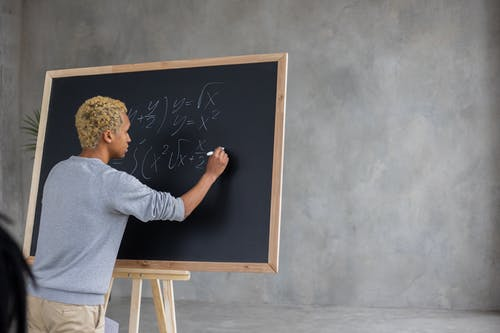## What Are Relations?

Most children probably already have an idea of what relations and functions are, but it’s always good to remind them. Moreover, it is not infrequent that kids confuse these two terms. So it’s always wise to start your lesson by providing a definition of relations and functions.

You can define a relation as a relationship between given sets of information and provide an example of a relation. For instance, ask students to think of all their peers in the class, their eye color, and hair color. The pairing of children’s eye color and hair color represents a relation.

Point out that the pairs of hair color and eye color are ordered or, in other words, we first write down the hair color and then the eye color (or vice versa). So in relations and functions, we’re talking about ordered sets of pairs (x, y).

You can now show students how to present relations: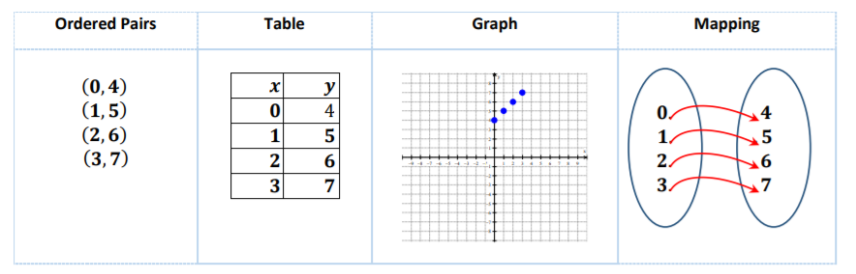## What Are Functions?

Point out that functions are a sub-category of relations – in other words, all functions are relations but not all relations are functions. You can define a function as a relation where no two ordered pairs have the same first element.

In simple terms, a function is a relation that relates every element from one set with only one element from another set. However, make sure to point out that while one element from one set (x) can’t relate to more than one element from another set (y); two or more elements from one set (x) can relate to the same element from another set (y).

### Domain and Range

In Algebra 2, relations and functions lessons also require learning about the domain and range of a function. Explain that the domain is the set of values for x (i.e. the set of all starting points or first values of a function), whereas the range of the function is the set of values for y (i.e. the set of all ending points or second values of a function).

You can provide an example of how we identify the domain and range of a function. For instance, identify the domain and range in the following relation:

R = {(2, a), (4, b), (6, c), (8, d)}

Since the domain represents the set of first values of a function, we can easily identify the domain as {2, 4, 6, 8}.

The range on the other hand represents the second values of a function, so we can observe that the range of this function is {a, b, c, d}.

### How to Identify if a Relation Is a Function?

You can also show children how to identify whether a relation is a function. This is an area where they can easily get confused, so it’s useful to provide several examples until they feel confident about their functions’ knowledge.

Explain to students that a relation is a function if the first set (x) has a one-to-one and one-to-many relationship with the other set (y). No function can be many-to-one or many-to-many. You can provide relatable examples. Ask students if the relation of the teacher in the classroom to each student is a function? One to many, so yes.

So one way of identifying whether a relation is a function is simply by identifying whether all the input values (or Xs) are different. If there are two or more input values that are repeated in the relation, then it’s not a function.

One way of doing this is with the help of a graph (with the vertical line test). With this option, that is, the vertical line test, we see if it’s possible to draw a vertical line that intersects the graph more than once.

If it is impossible to draw such a line, that means that every x value relates to a single y value. In other words, if we can’t draw a vertical line that intersects the graph more than once, the relation is a function.

#### Example 1:

Show children how to check whether a relation is a function by using the vertical line test. Draw a graph of a relation on the whiteboard, for instance: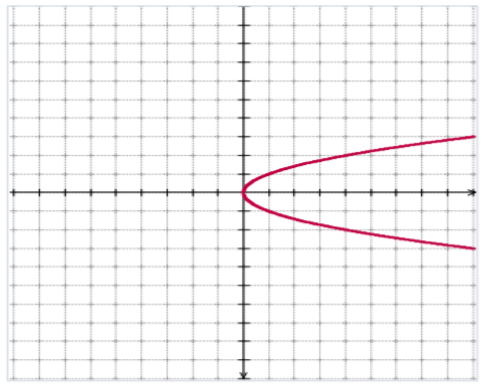Show students how we can draw a vertical line (x = 5) that would intersect the graph. Point out that since this vertical line crosses the graph in two points, this relation does not represent a function. This means that there is an x value that relates to more than one y value: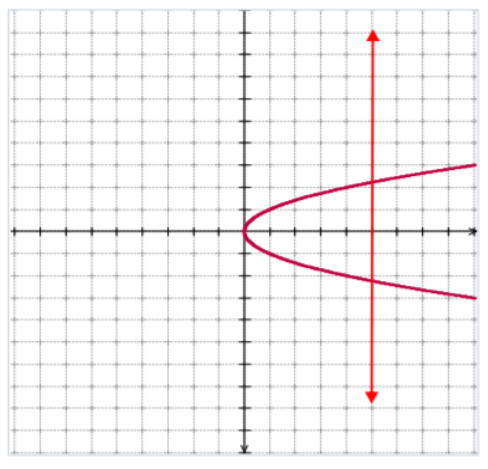#### Example 2:

Show children how we can determine whether the following relation is a function:

R = {(1, 5), (1, 6), (2, 9), (6, 9)}

If it helps them, you can either first determine the domain and range, or simply use a highlighter to highlight the x values in one color and the y values in another color.

In this relation, we can observe that there is a repetition of x values or input values, that is, it’s easy to notice that the first value 1 has been repeated twice, i.e. in (1, 5) and (1, 6).

#### Example 3:

Now you can ask children to determine whether this relation represents a function:

R = {(1, 1), (2, 2), (3, 1), (4, 2), (5, 1), (6, 7)}

By examining the input values, students should be able to notice that this relation represents a function, as there is no repetition of x values and no two ordered pairs have the same first element.

You can also introduce this lesson with the help of multimedia material, such as videos. Videos are a fun and engaging way to help children learn and can greatly enrich any math lesson. In addition, they’re a great resource for homeschooling parents who can pause and repeat a segment as many times as needed.

You can use this video that explains how to determine if a relation is a function by examining the input values or x values of the given relation. This video shows how to check whether a relation is a function with the help of a graph. Finally, this one focuses on recognizing functions from a graph.

Finally, you may also want to check out our bundle of free resources on analyzing graphs of functions and relations, which you can use once children are comfortable with arranging and identifying relations and functions.

## Activities to Practice Relations and Functions

### Mapping Diagrams Game

This activity will help students practice determining whether a relation is a function. To use this game in your classroom, you’ll need construction paper, paper (or cotton lines), paper numbers, glue, and scissors. You’ll need to prepare the circles, lines, and numbers.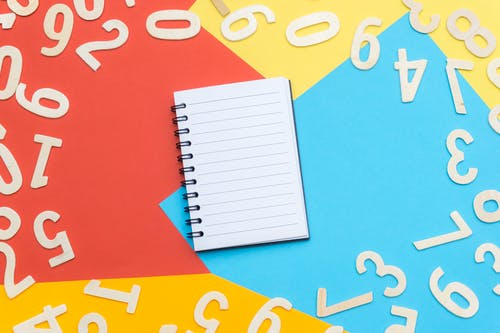The number of diagrams will depend on the number of students in your class and consequently, the number of groups. You’ll need to have one diagram per group, composed of two circles and lines connecting the numbers in them.

You can prepare the diagrams by cutting out two large circles from the construction paper. In addition, cut out several numbers from the construction paper, as well as a number of lines. It’s advisable that the numbers and circles are in different colors so that they add to the visual appeal of the activity.

#### Launch the Game!

Divide students into groups of 3 or 4. Each group should get two circles (circle X and circle Y), numbers, paper lines, and glue. Provide the instructions for the game to the groups. All members of a given group work together to complete their diagram.

Each group is supposed to glue the numbers they obtained in the two circles and connect them with the paper lines so as to form a function. They should pair up as many numbers from one circle to numbers from the other circle as possible, making sure that they obtain a function.

One caveat though: students can only place 3 numbers in circle Y. You can give 5 minutes to each group to do this. Just make sure that there is an equal amount of numbers and repeating numbers in each group, so that the game is fair for everyone.

The group that manages to use up most of its numbers and create the biggest number of ordered pairs that have a function wins the game. They could also explain why they paired the numbers from circle X to numbers of circle Y the way they did in front of the entire class.

### Domain and Range Game

Use this online game after you’ve shown the children how to identify the domain and range of functions. You can implement the game at the end of the lesson to further reinforce students’ knowledge of domain and range.

The game is played individually, which also makes it suitable for parents who are homeschooling their children. Students are asked to identify the range or the domain of a given relation. The best of all? They’re also provided with a helpful example if they get stuck at a question, which makes this game ideal for self-improvement.

### Identify Functions Game

This is an online game that will help children improve their skills at identifying functions. To play this game in your classroom, make sure that your students are already familiar with how to check whether a relation is a function. Ideally, they should also be familiar with the vertical line test.

Children are presented with different relations on a graph and are asked to determine whether these relations are functions. If they answer correctly, they score points and if they answer incorrectly, the game shows them what the correct answer is, as well as an explanation of why that is the correct answer.

## Before You Leave…

If you liked these tips and activities for Algebra 2 (relations and functions) we have a whole lesson with worksheets and fun resources on teaching this topic. So why not sign up for our emails for more free lessons and content!

In addition, you can sign up for a membership on MathTeacherCoach or head over to our blog, where you’ll find more math materials for kids of all ages. You’ll discover that with the resources we offer, teaching math is super fun and easy!